#Method of Variation of ParametersThis method is interesting whenever the previous method does not apply (when g(x) is not of the desired form). The general idea is similar to what we did for second order linear equations except that, in that case, we were dealing with a small system and here we may be dealing with a bigger one (depending on the order of the differential equation). Let us describe the general case (constant coefficients or not). Consider the equation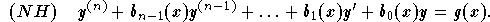Suppose that a set of independent solutions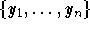of the associated homogeneous equation is known. Then a particular solution can be found as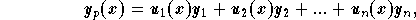where the functions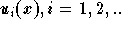can be obtained from the following system: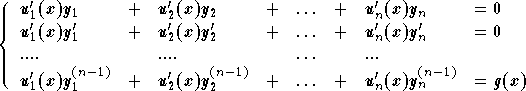.

The determinant of this system is the Wronskian of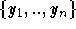, which is not zero. Cramer's formulas will give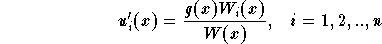,

where W(x) is the Wronskian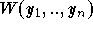and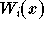is the determinant obtained from the Wronskian W by replacing the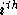-column in the vector column (0,0,..,0,1). Consequently, a particular solution to the equation (NH) is given by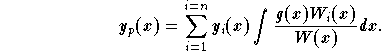Note that when the order of the equation is not high, you may want to solve the system using techniques other than Cramer's formulas.

Example: Find a particular solution of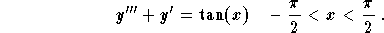Solution: Let us follow these steps:

(1)
Characteristic equation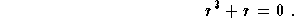Since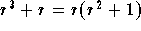, the roots of the characteristic equation are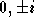. Therefore, a set of independent solutions is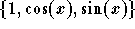;

(2)
A particular solution is given by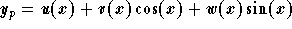, where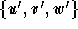are solutions of the system;

(3)
The resolution of the system gives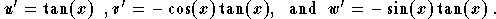After integration we get;

(4)
A particular solution is given by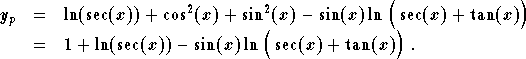Note that the constant 1 in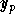may be dropped since it is the solution of the associated homogeneous equation.[Differential Equations] [First Order D.E.] [Second Order D.E.]
[Geometry] [Algebra] [Trigonometry ]
[Calculus] [Complex Variables] [Matrix Algebra]S.O.S MATHematics home page

Do you need more help? Please post your question on our S.O.S. Mathematics CyberBoard.Author: Mohamed Amine Khamsi